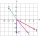Vector + scalar product - examples

1. Find the 10Find the value of t if 2tx+5y-6=0 and 5x-4y+8=0 are perpendicular, parallel, what angle does each of the lines make with the x-axis, find the angle between the lines?
2. TriangleTriangle KLM is given by plane coordinates of vertices: K[-12, -11] L[-15, -18] M[-13, -12]. Calculate its area and itsinterior angles.
3. Angle between vectorsFind the angle between the given vectors to the nearest tenth of a degree. u = (-22, 11) and v = (16, 20)
4. CuboidsTwo separate cuboids with different orientation in space. Determine the angle between them, knowing the direction cosine matrix for each separate cuboid. u1=(0.62955056, 0.094432584, 0.77119944) u2=(0.14484653, 0.9208101, 0.36211633)
5. Find the 5Find the equation with center at (1,20) which touches the line 8x+5y-19=0
6. Three points 2The three points A(3, 8), B(6, 2) and C(10, 2). The point D is such that the line DA is perpendicular to AB and DC is parallel to AB. Calculate the coordinates of D.
7. Scalar productCalculate the scalar product of two vectors: (2.5) (-1, -4)

We apologize, but in this category are not a lot of examples.
Do you have an interesting mathematical example that you can't solve it? Enter it, and we can try to solve it.

To this e-mail address, we will reply solution; solved examples are also published here. Please enter e-mail correctly and check whether you don't have a full mailbox.

Two vectors given by its magnitudes and by included angle can be added by our vector sum calculator.